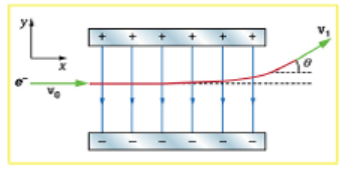# Problem: Figure 18.57 shows an electron passing between two charged metal plates that create an 100 N/C vertical electric field perpendicular to the electron's original horizontal velocity. (These can be used to change the electron's direction, such as in an oscilloscope.) The initial speed of the electron is 3.00×106 m/s, and the horizontal distance it travels in the uniform field is 4.00 cm. (a) What is the vertical deflection?(b) What is the vertical component of its final velocity? (c) At what angle does it exit? Neglect any edge effects.

###### FREE Expert Solution

The 4 UAM equations are:

Acceleration,

$\overline{){{\mathbit{a}}}_{{\mathbit{y}}}{\mathbf{=}}\frac{\mathbf{qE}}{\mathbf{m}}}$

Time,

$\overline{){\mathbf{t}}{\mathbf{=}}\frac{{\mathbf{L}}_{\mathbf{x}}}{{\mathbf{v}}_{\mathbf{0}\mathbf{x}}}}$

(a)

$\overline{){\mathbf{1}}{\mathbf{N}}{\mathbf{=}}{\mathbf{1}}{\mathbf{k}}{\mathbf{g}}{\mathbf{·}}{\mathbf{m}}{\mathbf{/}}{{\mathbf{s}}}^{{\mathbf{2}}}}$

97% (198 ratings)###### Problem Details

Figure 18.57 shows an electron passing between two charged metal plates that create an 100 N/C vertical electric field perpendicular to the electron's original horizontal velocity. (These can be used to change the electron's direction, such as in an oscilloscope.) The initial speed of the electron is 3.00×106 m/s, and the horizontal distance it travels in the uniform field is 4.00 cm.

(a) What is the vertical deflection?

(b) What is the vertical component of its final velocity?

(c) At what angle does it exit? Neglect any edge effects.